### Home > ACC6 > Chapter 6 Unit 6 > Lesson CC1: 6.2.4 > Problem6-106

6-106.

Find the perimeter and area of each figure made of algebra tiles below.

1.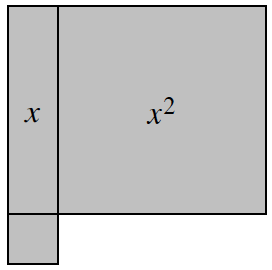Remember, the small square is a unit tile, which is a $1 × 1$ square.

The dimensions have been placed on the algebra tiles for you.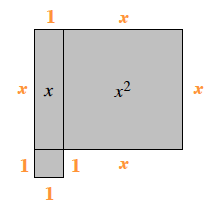$4x + 4$ units

1.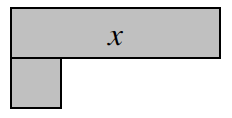Looking at the algebra tiles below, do you understand why the length of one side is labeled $x − 1$?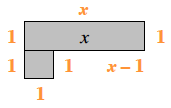Since part of the side with length $x$ touches the unit tile, the side that is included in the calculation of the perimeter is $x − 1$.

1.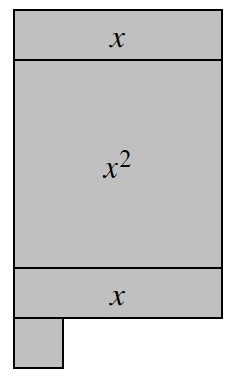Refer to parts (a) and (b).